# Number Series

Number series is a sequential arrangement of numbers following a certain defined pattern.

In this section, we deal with questions in which a series of numbers, (which are generally called the terms of the series) is given. These numbers/terms follow a certain pattern throughout the series. Candidates are asked either to find a missing term or to find the wrong term of the series.

## Important Patterns of the Series

Different series based on some particular patterns are discussed below

I. Based on Addition/Subtraction of Numbers

1. Addition/subtraction of consecutive odd/even numbers These series follow the pattern of addition or subtraction of even or odd numbers.

Example 1: 5, 6, 9, 14, 21, ?

a. 26   b. 28   c. 30   d. 34

Solution:  (c) Pattern of the series is as shown below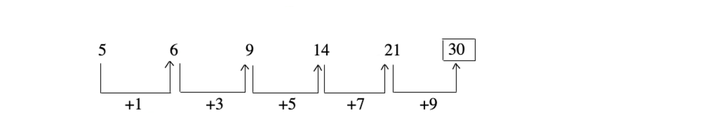The series consists of a pattern of addition of consecutive odd numbers.

Example 2: 40, 30, 22, 16, ?

a. 10          b. 12

c. 15         d. 17

Solution:  (b) Pattern of the series is as shown below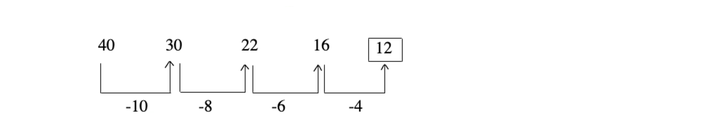The series consists of a pattern of subtraction of consecutive even numbers.

2. Addition/subtraction of prime numbers These series follow the pattern of addition/subtraction of prime numbers.

Example 3: 1, 3, 6, 11, 18, ?

a. 25       b. 29

c. 32       d. 35

Solution:  (b) Pattern of the series is as shown below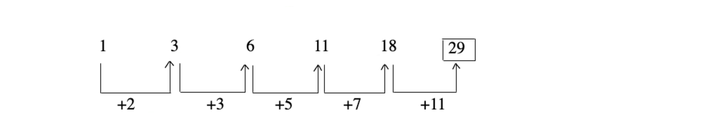The series consists of a pattern of addition of prime numbers.

Example 4: 32, 19, 8, ?

a. 12         b. 3

c. 1           d. 5

Solution:  (c) Pattern of the series is as shown below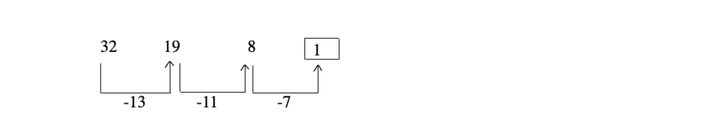The series consists of a pattern of subtraction of prime numbers.

II. Based on Multiplication or Division of Numbers

These series follow the pattern based on multiplication and division of numbers.

Example 5: 1, 3, 9, 27, 81, ?

a. 216      b. 220

c. 243      d. 250

Solution:  (c) Pattern of the series is as shown below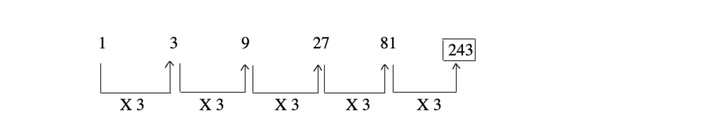Here, next number of this series is multiplication of 3 with previous one.

Example 6: 1296, 648, 216, ?, 36, 18, 6, 3

a.112           b. 108

c. 106          d. 110

Solution:  (b) Pattern of the series is as shown belowHere, the terms are divided by 2 and 3 alternately.

So, missing term is obtained as 216/2 = 108

III. Based on Addition/Subtraction of Squares/ Cubes of Natural Numbers

1. Additional/subtraction of squares of natural numbers These series follow the pattern based on addition/subtraction of squares of natural numbers.

Example 7: 1, 2, 6, 15, 31, ?

a. 56        b. 64

c. 60        d. 58

Solution:  (a) Pattern of the series is as shown below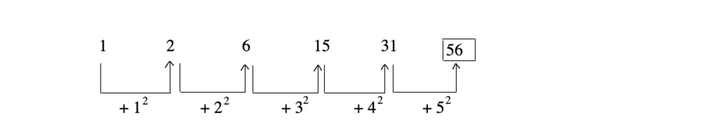The series is based on addition of squares of consecutive natural numbers.

Example 8: 71, 55, 46, 42, ?

a. 40       b. 41

c. 39       d. 38

Solution:  (b) Pattern of the series is as shown below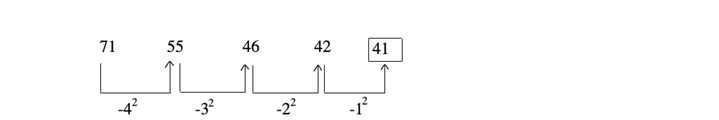The series is based on subtraction of squares of consecutive natural numbers.

2. Addition/subtraction of cube of natural numbers These series questions, follow the pattern based on addition/ subtraction of cubes of natural numbers.

Example 9: 1, 2, 10, 37, 101, ?

a. 402       b. 206

c. 226       d. 320

Solution:  (c) Pattern of the series is as shown below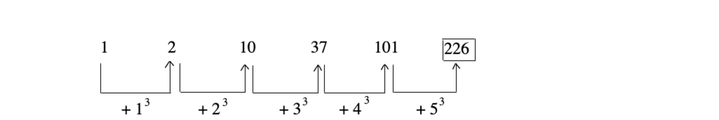The series is based on addition of cubes of consecutive natural numbers.

Example 10: 225, 100, 36, 9, 1, ?

a. 7             b. 6

c. 0             d. 1

Solution:  (c) Pattern of the series is as shown below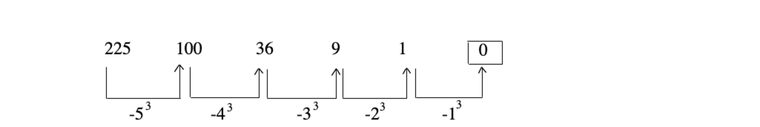The series is based on subtraction of cubes of consecutive natural numbers.

IV. Based on Multiple Operation

These series follow the pattern based on more than one mathematical operations. The multiple operations can be multiplication and addition, multiplication and subtraction, division and subtraction etc.

Example 11: 13, 25, 51, 101, 203, ?

a. 405             b. 406

c. 407             d. 411

Solution:  (a) Pattern of the series is as shown below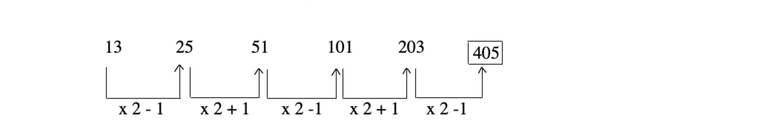The series is based on multiplication of 2 and then subtraction and addition of 1 alternately.

Example 12: 5, 11, 23, 47, 95, ?

a. 198             b. 194

c. 191             d. 185

Solution: (c) Pattern of the series is as shown below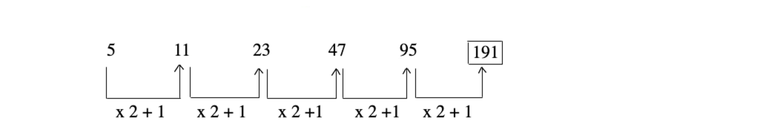The series is based on the pattern,
next number = (Previous number x 2 + 1)

V. Based on Combination of Two or More Series

These series contains the combination of two or more series.

Example 13: 1, 2, 2, 4, 3, 8, 7, 10, ?

a. 9               b. 8

c. 11             d. 13

Solution:  (c) Pattern of the series is as shown below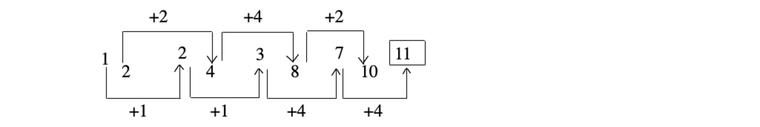This series contains two separate series. In first series, 1 is added in first two steps and 4 is added in third and fourth steps. In second series, 2 and 4 is added alternately.

Example 14: 3, 8, 6, 14, ?, 20

a. 12             b. 20

c. 42             d. 9

Solution:  (a) Pattern of the series is as shown belowThis sequence contains two separate series. In first series, each term is multiplied by 2.

In the second series, 6 is added to the previous term to obtain the next term.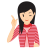Few Important things to Remember

• If the change is slow or gradual, then it is a difference series.

e.g.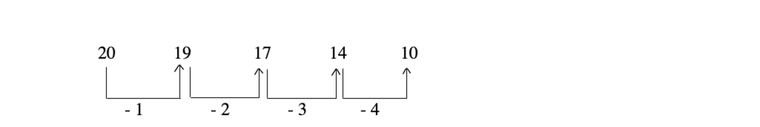• If the change is equally sharp, then it is ratio series.

e.g.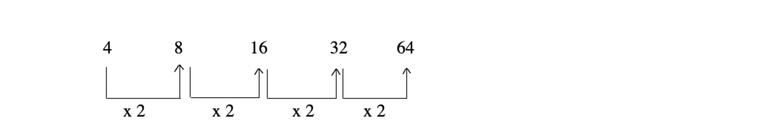• If the rise is sharp initially, but slows down later, then it is formed by adding squared, or cubed numbers.

e.g.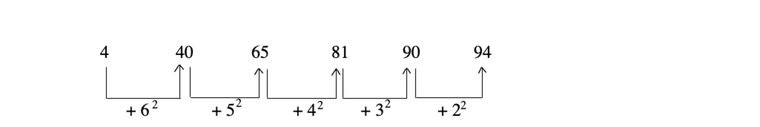• If the series is alternating, then it may be either a mixed series, or two different operations going on alternately.

e.g.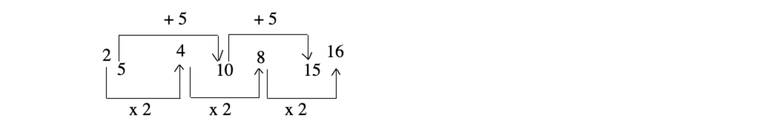## Type of Questions

There are mainly two types of questions which are asked in various competitive exams.

Type #1: To Find a Missing Term

Here, we are given a series of numbers including one or more missing numbers. This series follows a pattern, keeping this pattern in mind, we have to find the missing terms.

Direction (Example Nos. 15-17) In the following questions, a series is given with one term missing. Choose the correct alternative from the given ones that will complete the series.

Example 15: 831, 842, 853, 864, 875, ?

(a) 896                        (b) 876

(c) 886                        (d) 880

Solution:  (c) Pattern of the series is as shown below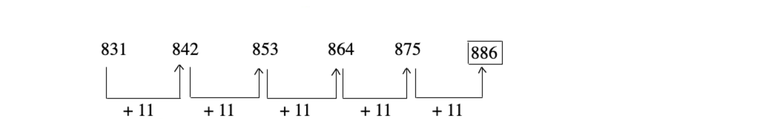Hence, the missing term is 886.

Example 16: 8, 16, ?, 64, 128

(a) 30                        (b) 32

(c) 28                        (d) 48

Solution:  (b) Pattern of the series is as shown below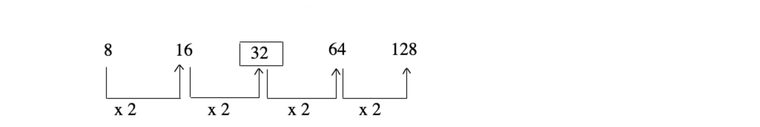Hence, the missing term is 32.

Example 17: 7, 8, 18, 57, ?, 1165

(a) 174                        (b) 232

(c) 224                        (d) 228

Solution:  (b) Pattern of the series is as shown below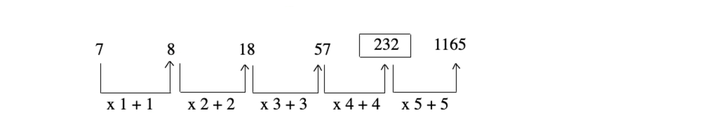Hence, the missing term is 232.

Type #2: To Find the Wrong term

Here, we are given a series of numbers.
Whole series except one number follow a certain rule. We have to find the number/term which does not follow the rule.

Direction (Example Nos. 18-19) In each of the following question, one number is wrong in the series. Find out the wrong number.

Example 18: 10, 14, 28, 32, 64, 68, 132

(a) 28                        (b) 32

(c) 64                        (d) 132

Solution:  (d) Pattern of the series is as shown below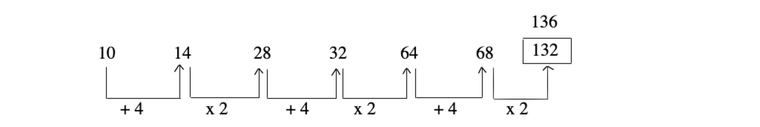So, 132 is wrong item, it must be replaced by
68 x 2 = 136

Example 19: 2, 3, 12, 37, 86, 166, 288

(a) 2                            (b) 3

(c) 166                        (d) 86

Solution:  (c) Pattern of the series is as shown below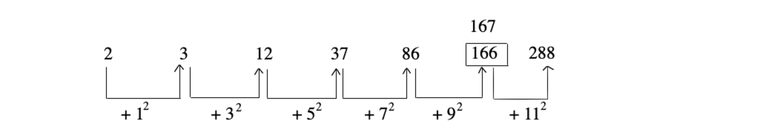So, 166 is wrong item, it must be replaced is 167.

## TIPS on cracking Reasoning Questions on Number Series

Tip #1: Arithmetic Series, Geometric Series, Patterns in Differences

(1) Arithmetic Series: When the differences between the successive numbers given in the series is the same. For example: 2, 5, 8, 11, 14... (Here the difference between the numbers is 3, hence the next number will be 17)

(2) Geometric Series: When each successive number in the series is obtained by multiplying or dividing the previous one by a fixed number. For example: 2, 6, 18, 72,…

(3) Patterns in differences: Calculate the differences between the numbers given in the series provided in the question. Then try to observe the pattern in the new set of numbers that you have obtained after taking out the difference.

Question: Look at this series: V, VIII, XI, XIV, __, XX, ... What number should fill the blank?

Solution:

Pattern of the series is as shown below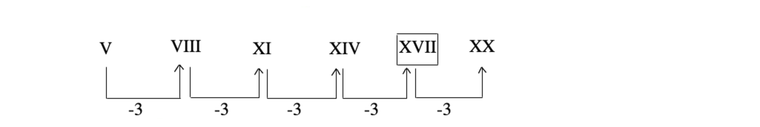This is an arithmetic series in Roman numerals; each no. is 3 more than the previous one. Thus, the missing number will be the Roman equivalent of 20 – 3 = 17, i.e., XVII.

Question: Look at this series: 23, 28, 34, 41, 49, ... What number should come next?

Solution:

Pattern of the series is as shown below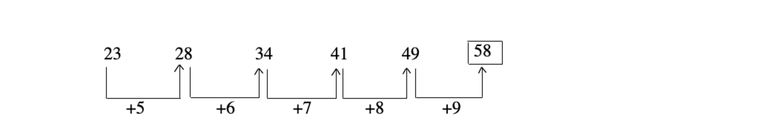The difference between the successive numbers is 5, 6, 7, 8 respectively. Thus, the difference keeps on increasing by 1. Thus, the next number in the series will be 49 + 9 = 58.

Tip #2: Pattern in Alternate/Adjacent numbers

(1) Pattern in Alternate numbers: When there is a pattern between every alternate or third number in the series. For example: 2, 9, 5, 12, 8 , 15, 11....

(2) Pattern in adjacent number: When adjacent numbers in the series changes based on a logical pattern. For example: 2, 4, 12, 48... Here the numbers are being multiplied by 2, then by 3, then by 4 etc.

Question: Look at this series: F2, __, D8, C16, B32, ... What number should fill the blank?

Solution:

This is a complex series in which the successive letters decrease by 1 and the successive numbers are multiplied by 2. Thus, the number in the blank will be E4.

Question: Look carefully at the following series and choose the pair that comes next.

42 40 38 35 33 31 28

A. 25, 22   B. 26, 23   C. 26, 24   D. 25, 23

Solution:

This is an alternating subtraction series in which 2 is subtracted twice, then 3 is subtracted once, then 2 is subtracted twice, and so on. So the next terms in the following series will be 26 and 24. Thus the correct answer is C.

Tip #3: Prime Numbers, Squares/Cubes, Alternate primes/exponents

(1) Squares/Cubes: When numbers are a series of perfect squares. For example: 9, 16, 25, 36, 49, 64...

(2) Cube/Square roots: When the numbers are a series of perfect cubes. For example: 27, 64, 125, 216, 343...

(3) Alternate Primes: Here the series is framed by taking the alternative prime numbers. For example: 2, 3, 5, 7…

Question: Look at this series: 4, 7, 16, 13, __, 19, 64, 29, ... What number should fill the blank?

Solution:

There are two alternating series here. The first one is the square of the multiples of 2 {22, 42, 62, 82 …} and the second one is a series of alternating prime numbers. The missing number is a part of the first series so the missing number is 62 = 36.

Question: Look carefully at the following series and choose the pair that comes next.

4  7  26  10 13 20 16

A. 14, 17   B. 18, 14   C. 19, 13   D. 19, 14

Solution:

Two patterns alternate here, with every third number following the alternate pattern. In the main series, beginning with 4, 3 is added to each number to arrive at the next. In the alternating series, beginning with 26, 6 is subtracted from each number to arrive at the next. So the next numbers will be 16 + 3 = 19, 20 – 6 = 14. Thus, the correct answer is D.

LearnFrenzy provides you lots of fully solved "Number Series " Logical Reasoning Questions and Answers with explanation.